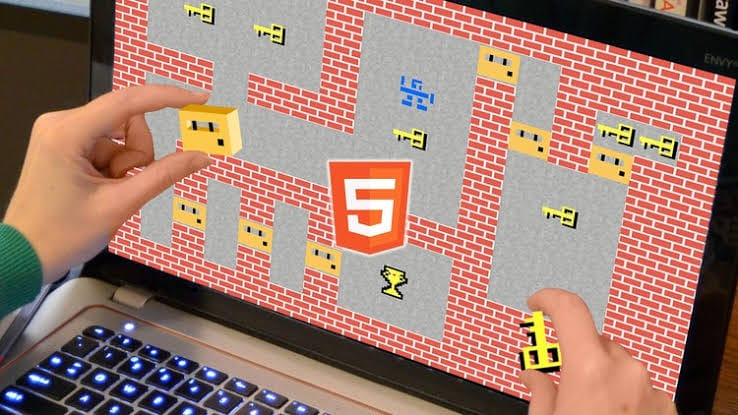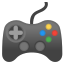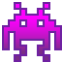# HTML Canvas Games from Scratch #1Hey there! This is my first blog about HTML Canvas Game Dev.
There are a lot of other tools and libraries available for game dev which are easier to use, but canvas remains my favorite as it takes us to the root of how to code game physics. It is also a great way for beginners to get a good grip on Javascript (speaking from experience).
Thanks to my friend Ronik Gandhi <@rawnix> for introducing me with canvas.

At the end of this series you will be able to build a basic 2D game on your own.

In this series I will walk you through the steps to build a classic Space Invadergame which I named SPACE-X.

It will look like this.

Do starmy repo (https://github.com/jrathod9/Space-X) if you liked the game.

Let's get started[Note: We will be creating games for pc only. They won't be responsive.]

## Basic Files and Boilerplate

``````📦Space-X
┣ 📂assets
┃ ┣ 📂Images
┃ ┗ 📂audio
┣ 📜index.html
┗ 📜code.js
``````

Get these folders and files ready. As of now we won't be using any assets, we will instead use javascript functions to create shapes.

This game without any images can be played [here] https://codepen.io/jrathod9/full/jXwMWQ

The index.html file will look something like :

```<!DOCTYPE html>
<html>
<title>Space-X</title>
<body>
<canvas id="canvas" style="background-color: black"></canvas>
</body>
<script type="text/javascript" src="code.js"></script>
</html>  ```

This index.html file consists of a canvas tag which is present inside the body tag.
There will be no more changes to this. Rest of the coding will be done in the code.js file.
The code.js file is linked after the closing body tag.

The code.js file will look something like:

```var canvas = document.querySelector('#canvas');
var c = canvas.getContext('2d');
canvas.width = window.innerWidth;
canvas.height = window.innerHeight;  ```
• The querySelector() method returns the first element that matches a specified CSS selector(s) in the document.
• The getContext() method returns an object that provides methods and properties to draw on the canvas. In this case since '2d' is mentioned, we can draw text, lines, rectangles, circles etc.
• Next we set the height and width of the canvas equal to the device window's height and width device (this can be changed according to your preference).

Now we are all set to begin coding the game!

## Phase 1

In this phase we will be working with particles and particle physics.
It is important to keep this in mind that the coordinate system of the canvas is laid down such that the origin is at top left corner of the screen :Before getting your hands dirty, these are some important methods you should be familiar with to draw on a canvas:

```c.clearRect(x1,y1,x2,y2); //clears the canvas inside this rectangular area
c.beginPath(); //Used to begin drawing a shape
c.closePath(); //Used to finish drawing a shape
c.fillStyle = 'red'; //Defines color to be filled in the shapes
c.fillStyle = '#ffffff'; //rgb,rgba and hex formats are also allowed
c.fillStyle = 'rgb(12,243,32)';
c.fillStyle = 'rgba(233,12,32,0.4)';//'a' is used to define opacity
c.fill(); //Fills color
c.strokeStyle = 'red'; ` //Defines stroke color (rgb,rgba,hex)
c.stroke(); //Strokes the boundary or the figure
c.font = "50px Calibri"; //Defines font properties of text
c.fillText("text" , x, y); //Writes text,top left of text is at (x,y)
c.arc(centerx,centery,radius, //Creates an arc with given properties
counterclockwise true or false);
c.moveTo(x,y); //Moves context cursor to (x,y)
c.lineTo(x,y); //Draws line from current context cursor coordinate to (x,y)  ```

NOTE : It is better to use beginPath() and closePath() separately for each connected figure.

A few sample code snippets: Code Link

Location in repository: \Phase 1\Sample CodeTry playing around with the code a little to get a better understanding of the working.
Also it takes time to get used to the syntax of these functions, so hands-on practice is the only solution.

Now let us try to code a particle in canvas.
Consider a particle object in a two dimensional plane. It will have properties:

• X Coordinate
• Y Coordinate

It is considered that the particle is a circle.
This is how we can represent the same in javascript :

```var particle = function(x,y,radius){
this.x = x;
this.y = y;
//'this' refers to the owner object, i.e. an instance of particle
}  ```

The above code defines an object type which is like a datatype , specifically it is a user-defined datatype. That means, now we can create variables of this type.
Lets create one named "atom".

`var atom = new particle(100,100,30);  `

This line creates a particle which can be referred with the variable "atom". It has the coordinates (100,100) and its radius is 50, but we still cannot see it on the canvas.

Note : All quantities are to be considered in 'pixels'.

Let us bring it to life by drawing it.

```c.beginPath();
c.fillStyle = 'aqua';
c.closePath();
c.fill();  ```

It is now drawn on the canvas. But now what if you want to set it in motion let us say to the right ?
You need a continuous loop in which:

• Canvas is cleared
• X coordinate of atom is incremented
• Atom is re-rendered on the canvas

The continuous loop is generated using the requestAnimationFrame() method.
The requestAnimationFrame() method calls the function, which is passed as a parameter, 60 times in one second. So now, we need a function for repetitive calling. Let us call this function 'draw' :

```var xspeed = 1; //Define x direction speed
function draw(){
//Clears the entire canvas
c.clearRect(0,0,window.innerWidth,window.innerHeight);
//Update x coordinate
atom.x += speed;
//Drawing the particle
c.beginPath();
c.fillStyle = 'aqua';
c.closePath();
c.fill();
requestAnimationFrame(draw); //Called inside the function
}
draw(); //Initial function call  ```

Result :In every consecutive function call, x coordinate of atom is incremented by the value of xspeed variable. To increase the speed, increase the value of xspeed.
Here is the source code : Code link

Location in repository : \Phase 1\Atom Particle

Similarly if you introduce a variable yspeed, which updates the y coordinate of atom, it will lead to a uniform straight line motion in the 2d plane.

```...
...
var yspeed = 2;
function draw(){
atom.y += yspeed;
...
...
}
draw();  ```

Result:### Javascript Math.random() function :

This deserves a separate section as it is very important to understand the working of the random function and how to control it. This function will be used very often in games for example:

• To spawn new enemies at random locations
• To spawn random powerups at random locations
• To give random moving directions to objects etc.

#### Syntax:

`var x = Math.random();  `

x gets assigned a random float value between 0 and 1 .

Note: value is inclusive of 0 but not 1.

Few outputs of the random function:

• 0.1882848343757757
• 0.3605824495503056
• 0.04217502958085739

How to get a random number between 0 and 1000?

`var x = Math.random()*1000;  `

This still gives a float value. For integer values:

```var x = Math.ceil(Math.random()*1000);
//Output: integer between 0 to 1000 both inclusive  ```

Math.ceil() function rounds a number up to the next largest whole number or integer.
There is another function called Math.floor() which returns the largest integer less than or equal to a given number.

Note : Lower bound is still 0.

How to get a random number between 500 and 1000?

`var x = Math.ceil(Math.random()*500) + 500;  `

Here initially Math.ceil(Math.random()*500) function returns values between {0,500} , thus on adding 500 to this range we get the new range {500,1000}.

How to get a negative range lets say -250 to 350?

`var x = Math.ceil(Math.random()*500) - 250;  `

If you aren't able to figure out how, try finding individual outputs of all the functions in the code one at a time.

This is all for this blog, in the next blog we shall see:

• How to handle multiple particles
• Random function in action
• Collisions
• Controlling the objects through user input

Written by : Jay Rathod